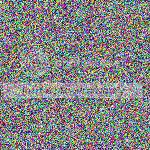# 如何使用PHP制作嘈杂的背景图像？

I'm looking to make an image that's just noise, maybe something like this:

Ideally I'd like to be able to change the colour as well. Any ideas on how to generate this?

• 点赞
• 写回答
• 关注问题
• 收藏
• 复制链接分享
• 邀请回答

#### 2条回答

• It's fairly straightforward to generate random noise. You can accomplish this pretty easily with some of PHP's image libraries, including the GD functions. I'm sure it would be similar in ImageMagick.

If you wanted to generate completely random noise, you could use random values for every color and every pixel. That might look something like this with GD:

``````//random colored noise
\$x = 150;
\$y = 150;
\$im = imagecreatetruecolor(\$x,\$y);
for(\$i = 0; \$i < \$x; \$i++) {
for(\$j = 0; \$j < \$y; \$j++) {
\$color = imagecolorallocate(\$im, rand(0,255), rand(0,255), rand(0,255));
imagesetpixel(\$im, \$i, \$j, \$color);
}
}
header('Content-Type: image/png');
imagepng(\$im);
``````

Generates this:However, the example image that you posted clearly doesn't look like completely random color noise. It seems more like an arbitrary choice between one of two colors, either a somewhat-grey pixel or a somewhat-colored pixel. You could accomplish that more like this:

``````//two-color random noise
\$x = 150;
\$y = 150;
\$im = imagecreatetruecolor(\$x,\$y);
\$color1 = imagecolorallocate(\$im, 200, 240, 242);
\$color2 = imagecolorallocate(\$im,220,220,220);
imagefill(\$im,0,0,\$color1);
for(\$i = 0; \$i < \$x; \$i++) {
for(\$j = 0; \$j < \$y; \$j++) {
if (mt_rand(0,1) == 1) imagesetpixel(\$im, \$i, \$j, \$color2);
}
}
header('Content-Type: image/png');
imagepng(\$im);
``````

Generates this:Your example seems a bit more complex still, with the pixels seeming to appear in small groups to produce a blockier appearance. You could emulate that by adjusting the loop logic if you wanted, or coloring small squares instead of individual pixels.

An interesting thing about this type of generation is that you can actually see the breakdown of the `rand()` function on Windows platforms if you use it instead of `mt_rand()`. Discernible patterns can develop in the noise due to limitations in that function/platform combination.

点赞 评论 复制链接分享
• Yes, you need to use:

• `ImageCreate[True]Color()`
• 2 x `for` loops
• `rand()` or `mt_rand()`
• `ImageColorAllocate()`
• `ImageSetPixel`

Sounds like a recipe, lol. Let me know if you need further help.

Sorry for the brevity had to do something, here is a sample code:

``````<?php

\$im = ImageCreateTrueColor(200, 300);

if (is_resource(\$im)) {
\$blue = array_map('hexdec', str_split('0000FF', 2));
\$white = array_map('hexdec', str_split('FFFFFF', 2));

\$blue = ImageColorAllocate(\$im, \$blue, \$blue, \$blue);
\$white = ImageColorAllocate(\$im, \$white, \$white, \$white);

for (\$w = 1; \$w <= 200; \$w++) {
for (\$h = 1; \$h <= 300; \$h++) {
if (mt_rand(1, 100) >= 50)
ImageSetPixel(\$im, \$w, \$h, \$blue);
else
ImageSetPixel(\$im, \$w, \$h, \$white);
}
}
}

header('Content-type: image/png');

ImagePNG(\$im, null, 9);
ImageDestroy(\$im);

?>
``````
点赞 评论 复制链接分享